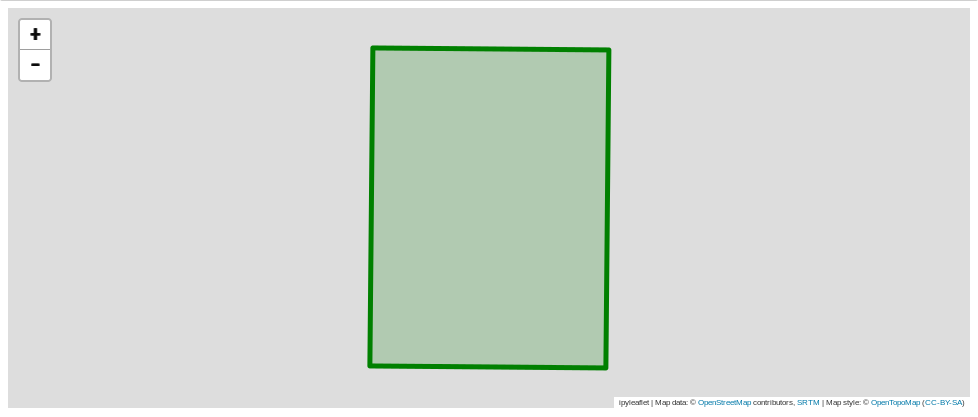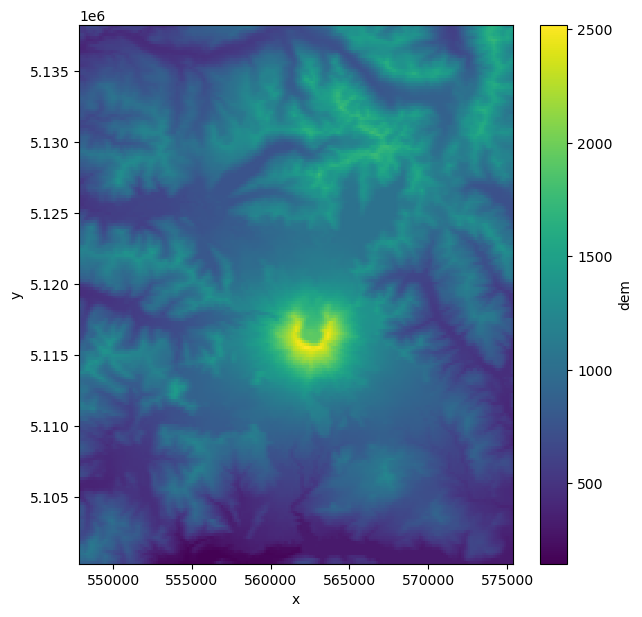Version:This tutorial is presented as Python code running inside a Jupyter Notebook, the recommended way to use Salvus. To run it yourself you can copy/type each individual cell or directly download the full notebook, including all required files.# Surface topographyThe effects of free-surface topography on seismic waveforms are significant, and any local study considering higher-frequency data must take topography into account. In this tutorial we'll explore how to add realistic free-surface topography to a SimulationConfiguration object, and investigate how high-order accurate topography / material interpolation can be used to improve simulation performance. To accomplish this, we'll focus on a real-life use case using the area around Mt. St. Helens in Washington state as an example. Of course please feel free to adapt the geographical area.
As always, we'll start by importing the Salvus namespace.
Copy
%matplotlib inline
import os
import pathlib
import salvus.namespace as sn
# This notebook will use this variable to determine which
# remote site to run on.
SALVUS_FLOW_SITE_NAME = os.environ.get("SITE_NAME", "local")
PROJECT_DIR = "project"

## Getting topography files

There are a number of ways topography can be specified in Salvus. In addition it has two built-in ways to acquire real world digital elevation data at fairly high resolution:
1. Via the GMRT web service
2. Via the AppEEARS web service
We focus on the first approach for the purpose of this tutorial. At the end there is an appendix to demonstrate how to use data acquired via the AppEEARs web service.

### Using the GMRT web service

The first step is to define the geographical domain of interest. A convenient way to do this is to use the specialized UtmDomain.from_spherical_chunk constructor that takes WGS84 coordinates and converts them to an appropriate UTM domain. The UTM zone and coordinates could of course also be specified directly.
d = sn.domain.dim3.UtmDomain.from_spherical_chunk(
min_latitude=46.15,
max_latitude=46.30,
min_longitude=-122.28,
max_longitude=-122.12,
)

# Have a look at the domain to make sure it is correct.
d.plot()# Where to download topography data to.
topo_filename = "data/gmrt_topography.nc"

# Now query data from the GMRT web service.
filename=topo_filename,
# The buffer is useful for example when adding sponge layers
# for absorbing boundaries so we recommend to always use it.
buffer_in_degrees=0.1,
resolution="default",
)
# Load the data to Salvus compatible SurfaceTopography object. It will resample
# to a regular grid and convert to the UTM coordinates of the domain.
# This will later be added to the Salvus project.
t = sn.topography.cartesian.SurfaceTopography.from_gmrt_file(
name="st_helens_topo",
data=topo_filename,
resample_topo_nx=200,
# If the coordinate conversion is very slow, consider decimating.
decimate_topo_factor=5,
# Smooth if necessary.
gaussian_std_in_meters=0.0,
# Make sure to pass the correct UTM zone.
utm=d.utm,
)
The interface we just used above to read in topography data is very general and also works for other data formats, and we'll see in a future tutorial how to read in surface, Moho, or discontinuity topography using a similar simplified interface. As is true for the volumetric models used in Salvus, once the topography files are read and processed they are stored as xarray datasets in memory. These datasets are simple to manipulate and visualize, and we can for instance plot the digital elevation model in the notebook by simply plotting the xarray dataset.
t.ds.dem.T.plot(aspect=1, size=7)
<matplotlib.collections.QuadMesh at 0x7f61fd51c550>Looks like St. Helens to me!
We can now create/load the project as seen in many other tutorials.
# Uncomment the following line to delete a
# potentially existing project for a fresh start
# !rm -rf project
p = sn.Project.from_domain(path=PROJECT_DIR, domain=d, load_if_exists=True)

## Adding topography to a project

Adding the previously downloaded and parsed topography is as simple as adding the SurfaceTopography object to Salvus:
p += t
Salvus is now aware of this topography and it can be used for simulations.
p.entities.list("topography_model")
['st_helens_topo']

Now we'll add an explosive source and a line of receivers to the project. Here we use the SideSet source and receiver classes. The functionality of these classes is documented here. Essentially, they help when the actual surface of the domain is non-trivial, as is the case in this example. When these sources and receivers are associated with a mesh, a small optimization problem is solved to ensure that they are placed exactly at the surface of the deformed mesh, plus or minus any offset one might specify. Here we specify the entities in UTM coordinates, place the source 1000 meters below the free surface, and place a line of 50 receivers directly at the free surface.
# Src / Rec reference coordinates.
src_x, src_y, src_z = 562700.0, 5112500.0, 4000.0
rec_x, rec_y, rec_z = 564700.0, 5115500.0, 4000.0
# Place an explosive source 1000 m below the free surface.
src = sn.simple_config.source.cartesian.SideSetMomentTensorPoint3D(
point=(src_x, src_y, src_z),
direction=(0, 0, 1),
side_set_name="z1",
mxx=1e21,
myy=1e21,
mzz=1e21,
myz=0.0,
mxz=0.0,
mxy=0.0,
offset=-1000.0,
)
# Place a line of receivers at the free surface.
rec = [
point=(rec_x, rec_y + _i * 100, rec_z),
direction=(0, 0, 1),
side_set_name="z1",
fields=["velocity"],
station_code=f"XX_{_i}",
)
for _i in range(50)
]
# Add the event collection to the project.
p += sn.EventCollection.from_sources(sources=[src], receivers=rec)

## Initializing a simulation

The next few commands should now be familiar after the previous tutorials. Below we initialize an isotropic elastic background model, a homogeneous model configuration constructed from this background model and our event configuration. We could also consider adding a 1-D background model, or a 3-D material model, at this stage, but we'll save this for a future tutorial.
bm = sn.model.background.homogeneous.IsotropicElastic(
rho=2600.0, vp=3000.0, vs=1875.5
)
mc = sn.model.ModelConfiguration(background_model=bm)
ec = sn.EventConfiguration(
wavelet=sn.simple_config.stf.Ricker(center_frequency=1.0),
waveform_simulation_configuration=sn.WaveformSimulationConfiguration(
end_time_in_seconds=6.0
),
)
Below we do something new, but which follows the model API we're now familiar with. In the cell below we initialize a TopographyConfiguration object. As is true with the ModelConfiguration, this object takes as arguments one or more topography models. A background topography model does not have much of a meaning, so it is not defined for topography configurations.
tc = sn.topography.TopographyConfiguration(topography_models="st_helens_topo")
Next we initialize an object which will inform the extrusion of the domain for the absorbing boundaries.
ab = sn.AbsorbingBoundaryParameters(
reference_velocity=3000.0,
reference_frequency=5.0,
number_of_wavelengths=3.5,
)
And now we've got enough information to define our complete simulation configuration. We create the configuration below and add it to the project as usual.
p += sn.SimulationConfiguration(
name="sim_1st_order_topo",
tensor_order=1,
model_configuration=mc,
event_configuration=ec,
absorbing_boundaries=ab,
elements_per_wavelength=1,
max_depth_in_meters=2000.0,
max_frequency_in_hertz=2.0,
topography_configuration=tc,
)
We can now visualize the simulation mesh with our topography on top. Looks pretty fancy!
p.viz.nb.simulation_setup("sim_1st_order_topo", ["event_0000"])
[2023-05-29 19:43:36,151] INFO: Creating mesh. Hang on.